Home / Digital Circuits / Encoder and Decoder

# Encoder and Decoder

Want create site? Find Free WordPress Themes and plugins.

Data can be stored in the form of binary numbers. Depending on the number of digits available, the magnitude of information stored changes, but more information can always be stored with less data. Having 4 bits of storage (say, four flip-flops), for example, offers the possibility of 24 = 16 possible cases, not 4. The binary data are called code.

Code conversion refers to the translation between the data stored in bits and the data for an application, in other words, conversion of data from one form to another.

A good example is a conversion from four bits of information that are available on four lines lighting up 10 decimal numbers (from 0 to 9) on a seven-segment LED. Although four bits (lines) can hold up to 16 numbers, all 16 do not necessarily need to be utilized. A seven-segment LED has seven LED bars, but not all must be turned on. The code converter receives the inputs for a number to be displayed and depending on the input states (corresponding to the number) determines which bars must be lit.

## Decoder

In general, a binary code of N bits can represent 2N distinct values. A decoder is a combination circuit that converts the binary information from N inputs to a maximum of 2N distinct outputs. In a way, it extracts information from packaged data.

A decoder is a combination circuit meaning that it consists of various gates that put together a number of conditions carried by the input code. Figure 1 depicts the circuit for a decoder, which translates from BCD (binary-coded decimal) to decimal numbers. This circuit is good for lighting up one light out of 10 or opening a door out of 10 doors, for example, corresponding to each number. Comparing with 10 switches and 10 lines, the same function is performed with only four lines.

As another example consider a decoder that converts from binary digits to a seven-segment display to illuminate numbers 0 to 9, that is, for lighting up a one-digit decimal number.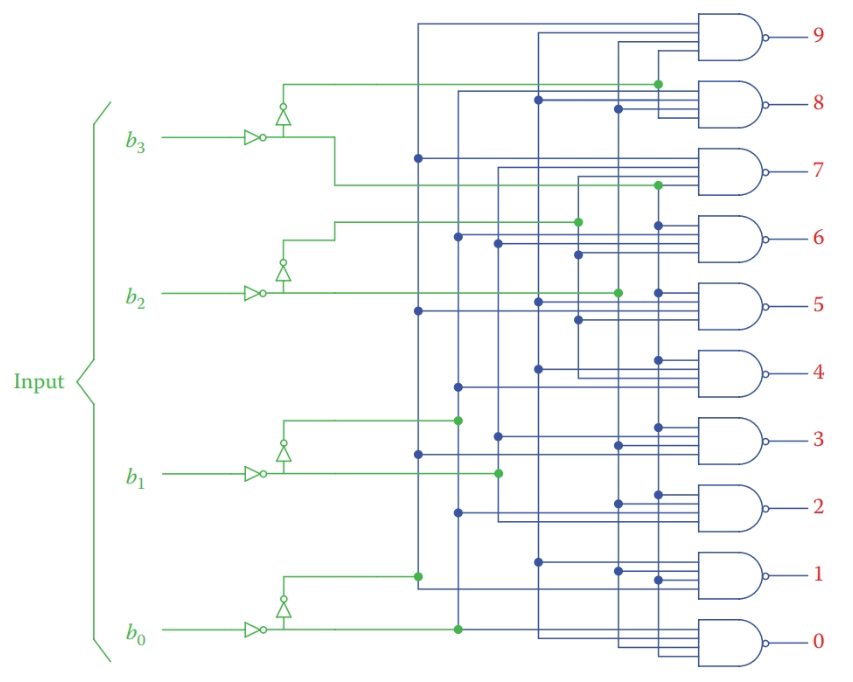Figure 1 Circuit for BCD to decimal decoder.

The decoder logic circuit is defined based on writing the requirement for each output element and simplifying the resulting logic expression. For example, the requirement for a decoder to light up a seven-segment display is as shown in Figure 2. For each single digit number, certain segments in the display must be lit. The segments are named a to g, as shown in the figure. Those that must be lit are tabulated for all digits from 0 to 9.

Study of the table in Figure 2 reveals that it shows the truth table of the decoder circuit. Each segment in the display (a to g) can be expressed as a function of the four inputs. A Karnaugh map can be used to simplify the logic expression.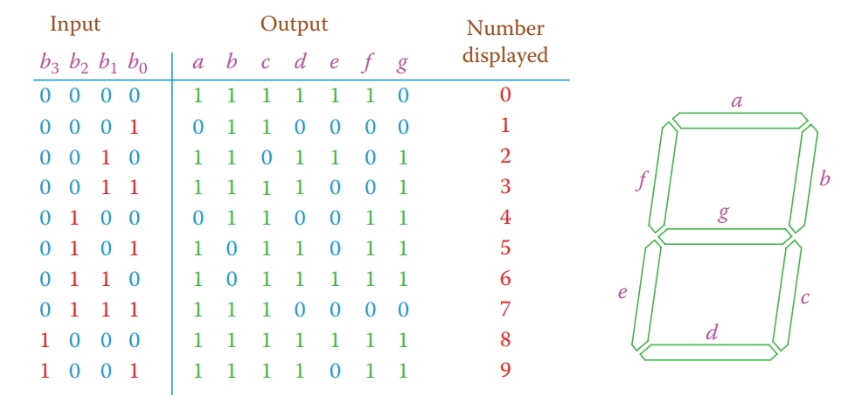Figure 2 Truth table for BCD to seven-segment display.

## Encoder

An encoder does the reverse of a decoder. It has 2N or less inputs containing information, which are converted to be held by N bits of output.

The best example of an encoder is what is used to measure the rpm of a rotating shaft or to find the angle position of a shaft in one revolution. The first one, called incremental encoder, can be used in an anemometer, a device that measures the wind speed. The second one, called absolute encoder, can be used in a wind vane to detect the wind direction.

There are plenty of applications of encoders in the industry and home devices. Without going into much detail we briefly study the encoder part of the second type.

This encoder generates a four- or five-digit binary code that can be transmitted with the same number (4 or 5) of wires carrying 0 and 1 values, which then can be decoded to find the angle position of a shaft within 360°.

The resolution of measurement depends on whether four or five (or other) number of digits are used. The more the number of digits (and the wires) the more accurate the reading is. For four digits, each reading corresponds to 360 ÷ 24 = 22.5°. For five digits, each reading corresponds to 360 ÷ 25 = 11.25°.

Incremental encoder: Type of encoder (for a rotating shaft) that adds the number of revolutions and determines the total displacement of a shaft during a given time. This is as opposed to an absolute encoder that determines the displacement (and position) of a shaft within only one revolution.

Anemometer: Device to measure the wind speed.

Absolute encoder: Determines the angular position of a shaft or rotating body in its 360° angular displacement as opposed to incremental encoder.

Wind vane: Instrument for detecting the direction of the wind, consisting of an aerodynamic body that rotates about an axis and can orient itself with the wind direction.

The physical part of the encoder consists of a flat round disk on which four or five tracks are painted. Each track is divided into a number of black and white segments and a sensor can detect if a segment is black or white, which stand for 0 and 1.

The arrangement of segments is such that each position of the disk (within the possible resolution) can be defined by a unique combination of 0’s and 1’s.

Figure 3 shows a disk with four tracks. Two types of segments are shown, one called binary code and the other gray code. With this type of encoder, the gray code (which uses a different code than the binary numbers) offers an advantage that makes it more desirable in an application.

The advantage of the gray code over the binary code is in that moving from one number to the next implies only one-bit change and not more than one. This allows detecting of any error if takes place in data reading and number processing.Figure 3 Tracks on a shaft encoder: (a) binary code and (b) gray code.

For better clarity and comparison of the binary code and gray code, the tracks containing black and white segments are shown straight (instead of round) in Figures 4 and 5.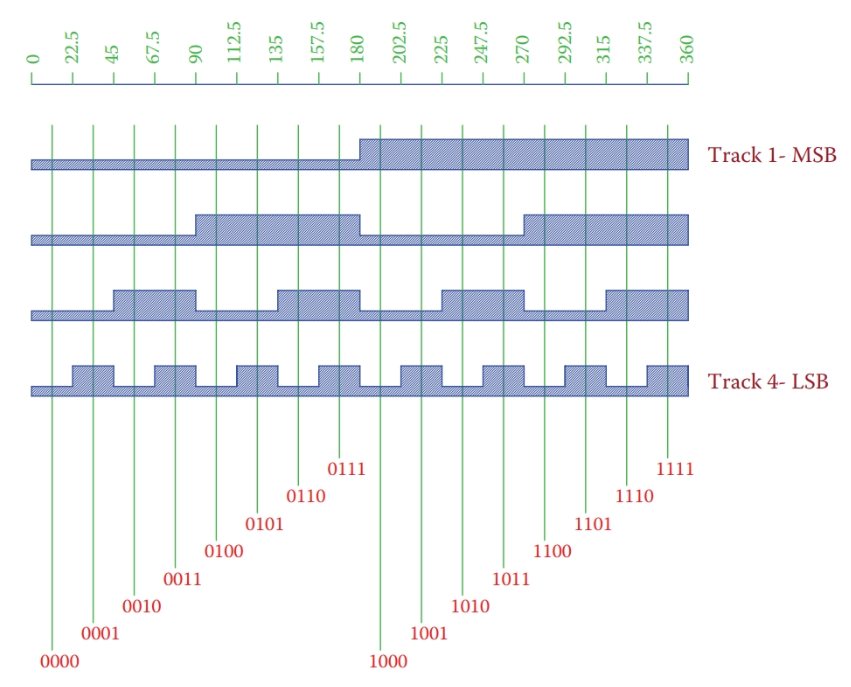Figure 4 Representation of binary code tracks on the disk of a shaft encoder.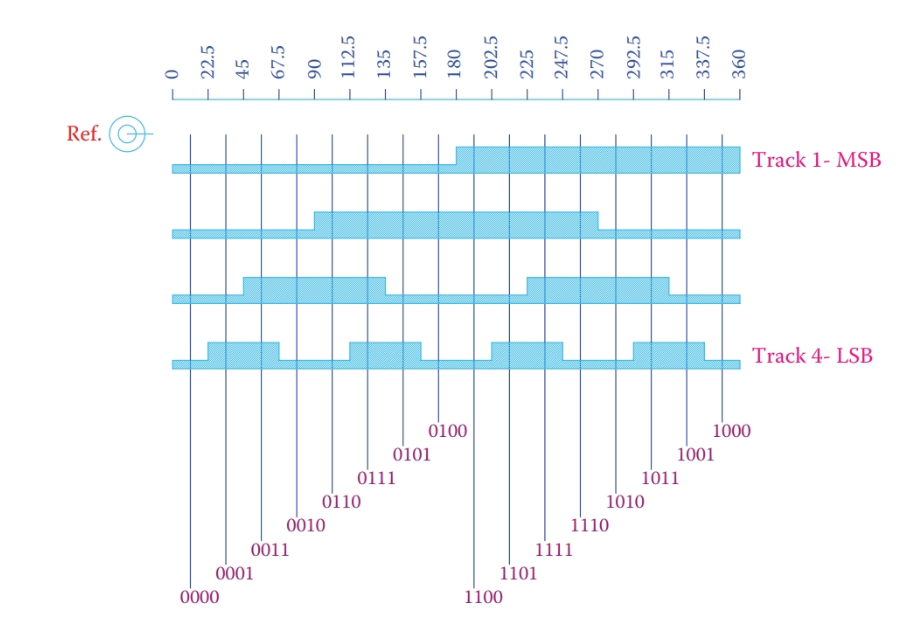Figure 5 Representation of gray code tracks on the disk of a shaft encoder.

Table 1 shows the difference between the binary code and the gray code.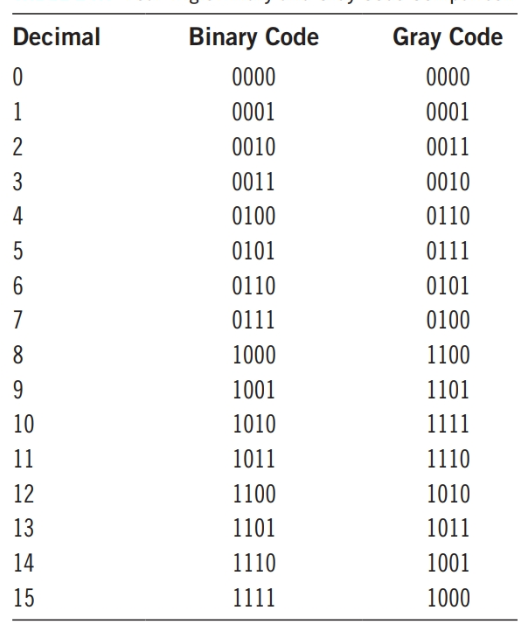Table 1 Four-Digit Binary and Gray Code Comparison

Did you find apk for android? You can find new Free Android Games and apps.